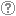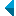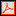# Search the repositoryQuery: search in TitleAuthorAbstractKeywordsFull textYear of publishing ANDORAND NOT search in TitleAuthorAbstractKeywordsFull textYear of publishing ANDORAND NOT search in TitleAuthorAbstractKeywordsFull textYear of publishing ANDORAND NOT search in TitleAuthorAbstractKeywordsFull textYear of publishing Work type: All work types Habilitation (m4) Specialist thesis (m3) High school thesis (m6) Bachelor work * (dip) Master disertations * (mag) Doctorate disertations * (dok) Research Data or Corpuses (data) * old and bologna study programme Language: All languagesSlovenianEnglishGermanCroatianSerbianBosnianBulgarianCzechFinnishFrenchGerman (Austria)HungarianItalianJapaneseLithuanianNorwegianPolishRussianSerbian (cyrillic)SlovakSpanishSwedishTurkishUnknown Search in: RUP    FAMNIT - Faculty of Mathematics, Science and Information Technologies    FHŠ - Faculty of Humanities    FM - Faculty of Management    FTŠ Turistica - Turistica – College of Tourism Portorož    FVZ - Faculty of Health Sciences    IAM - Andrej Marušič Institute    PEF - Faculty of Education    UPR - University of Primorska    ZUP - University of Primorska PressCOBISS    Fakulteta za humanistične študije, Koper    Fakulteta za management Koper in Pedagoška fakulteta Koper    Fakulteta za vede o zdravju, Izola    Knjižnica za tehniko, medicino in naravoslovje, Koper    Turistica, Portorož    Znanstveno-raziskovalno središče Koper Options: Show only hits with full text Reset

 1 - 5 / 511.Rank-permutable additive mappingsAleksandr Èmilevič Guterman, Anna A. Alieva, Bojan Kuzma, 2006, original scientific articleAbstract: Let ▫$\sigma$▫ be a fixed non-identical permutation on ▫$k$▫ elements. Additive bijections ▫$T$▫ on the matrix algebra ▫$M_n(\mathbb{F})$▫ over a field ▫$\mathbb{F}$▫ of characteristic zero, with the property that ▫$\rm{rk} (A_1...A_k) = \rm{rk} (A_{\sigma(1)}...A_{\sigma(k)})$▫ implies the same condition on the ▫$T$▫ images, are characterized. It is also shown that the surjectivity assumption can be relaxed, if this property is preserved in both directions.Found in: osebiKeywords: mathematics, linearna algebra, matrix algebra, rank, permutation, additive preserversPublished: 15.10.2013; Views: 2632; Downloads: 85Full text (0,00 KB) 2.Bar´ery Gibsona dlja problemy PoliaAleksandr Èmilevič Guterman, Gregor Dolinar, Bojan Kuzma, 2010, published scientific conference contributionAbstract: V članku je obravnavana spodnja meja za število neničelnih elementov v ▫$(0, 1)$▫ matrikah, pri katerem se da permanento vedno pretvoriti v determinanto samo s spreminjanjem predznaka ▫$pm$▫ elementom matrike.Found in: osebiKeywords: matematika, linearna algebra, teorija matrik, permanenta, determinantaPublished: 03.04.2017; Views: 1506; Downloads: 60Full text (0,00 KB)This document has more files! More... 3.Permanent versus determinant over a finite fieldAleksandr Èmilevič Guterman, Gregor Dolinar, Bojan Kuzma, Marko Orel, 2013, published scientific conference contributionAbstract: Let ▫$\mathbb{F}$▫ be a finite field of characteristic different from 2. We study the cardinality of sets of matrices with a given determinant or a given permanent for the set of Hermitian matrices ▫$\mathcal{H}_n(\mathbb{F})$▫ and for the whole matrix space ▫$M_n(\mathbb{F})$▫. It is known that for ▫$n = 2$▫, there are bijective linear maps ▫$\Phi$▫ on ▫$\mathcal{H}_n(\mathbb{F})$▫ and ▫$M_n(\mathbb{F})$▫ satisfying the condition per ▫$A = \det \Phi(A)$▫. As an application of the obtained results, we show that if ▫$n \ge 3$▫, then the situation is completely different and already for ▫$n = 3$▫, there is no pair ofmaps ▫$(\Phi, \phi)$▫, where ▫$\Phi$▫ is an arbitrary bijective map on matrices and ▫$\phi \colon \mathbb{F} \to \mathbb{F}$▫ is an arbitrary map such that per ▫$A = \phi(\det \Phi(A))$▫ for all matrices ▫$A$▫ from the spaces ▫$\mathcal{H}_n(\mathbb{F})$▫ and ▫$M_n(\mathbb{F})$▫, respectively. Moreover, for the space ▫$M_n(\mathbb{F})$▫, we show that such a pair of transformations does not exist also for an arbitrary ▫$n > 3$▫ if the field ▫$\mathbb{F}$▫ contains sufficiently many elements (depending on ▫$n$▫). Our results are illustrated by a number of examples.Found in: osebiKeywords: mathematics, linear algebra, matrix theory, permanent, determinantPublished: 03.04.2017; Views: 1536; Downloads: 85Full text (0,00 KB) 4.Commuting graphs and extremal centralizersGregor Dolinar, Aleksandr Èmilevič Guterman, Bojan Kuzma, Polona Oblak, 2014, original scientific articleAbstract: We determine the conditions for matrix centralizers which can guarantee the connectedness of the commuting graph for the full matrix algebra ▫$M_n(\mathbb{F})$▫ over an arbitrary field ▫$\mathbb{F}$▫. It is known that if ▫$\mathbb{F}$▫ is an algebraically closed field and ▫$n \ge 3$▫, then the diameter of the commuting graph of ▫$M_n(\mathbb{F})$▫ is always equal to four. We construct a concrete example showing that if ▫$\mathbb{F}$▫ is not algebraically closed, then the commuting graph of ▫$M_n(\mathbb{F})$▫ can be connected with the diameter at least five.Found in: osebiKeywords: commuting graph, matrix ring, centralizerPublished: 31.12.2021; Views: 264; Downloads: 19This document has more files! More... 5.Graph characterization of fully indecomposable nonconvertible (0, 1)-matrices with minimal number of onesMikhail Budrevich, Gregor Dolinar, Aleksandr Èmilevič Guterman, Bojan Kuzma, 2019, original scientific articleFound in: osebiKeywords: permanent, indecomposable matrices, graphsPublished: 03.01.2022; Views: 324; Downloads: 24Full text (278,69 KB)
Search done in 0 sec.